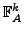# Hyper-digital and modal logic

may 2007

## Abstract

Recall the syntax of hyper-propositional logic (see e.g. ): Starting with any given atom set A we obtained an infinite hierarchy of formula setsby applying the following syntactical constructions:

• The box and diamond operator, that take a formula of degree k and return a formula of degree k+1.
• The usual conjunction, disjunction, and negation, that take their arguments fromand return a formula of the same degree k.
Let us write F(A) for the (mixed) hyper-(propositional) formula set, i.e. the union of all the(see )

On the other hand, there are the well known modal formulas M(A): given a set A, they are constructed by recursively and arbitrarily applying the box, diamond, conjunction, disjunction, and negation. But different to F(A), there are no degrees, the constructions can be performed on any argument formulas. We can read hyper-formulas as modal formulas. In this respect, F(A) is a subset of M(A).

Of course, the semantics of the two systems are very different. Hyper-formulas are interpreted as bit tables, modal formulas on the other hand describe Kripke models. Our goal here is to investigate the possible relations and mutual translations of these two systems.

## The text

... is under construction ...